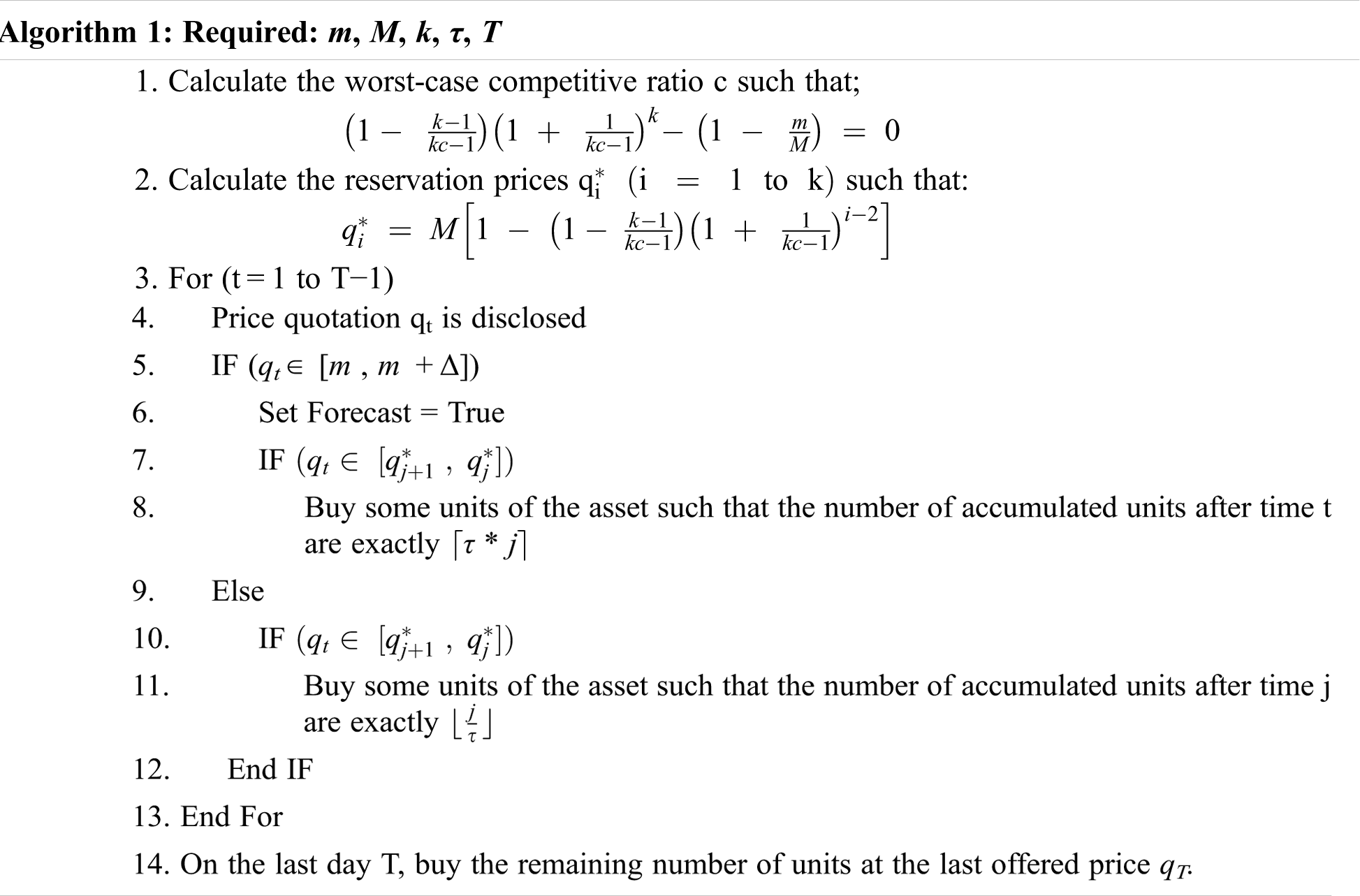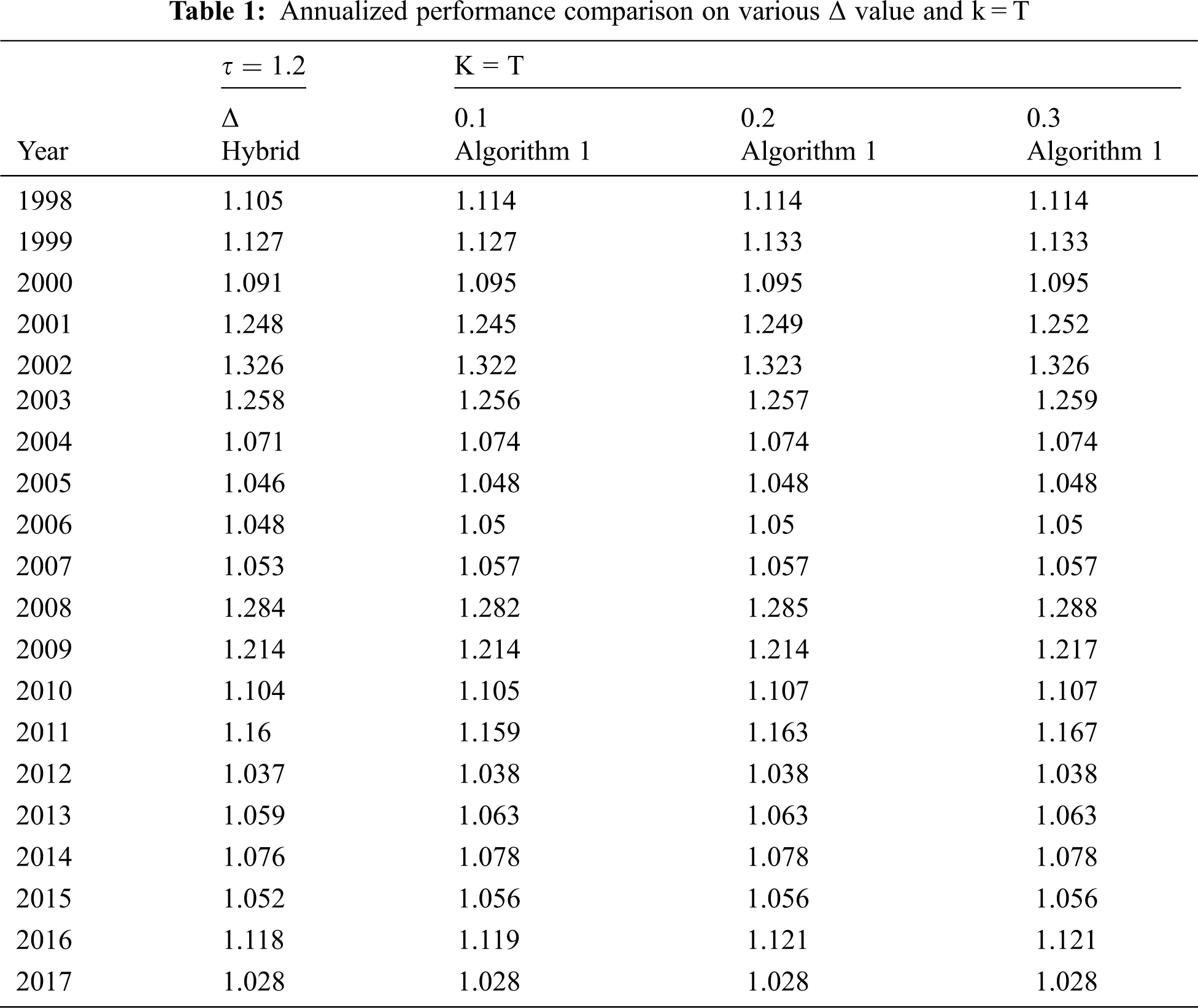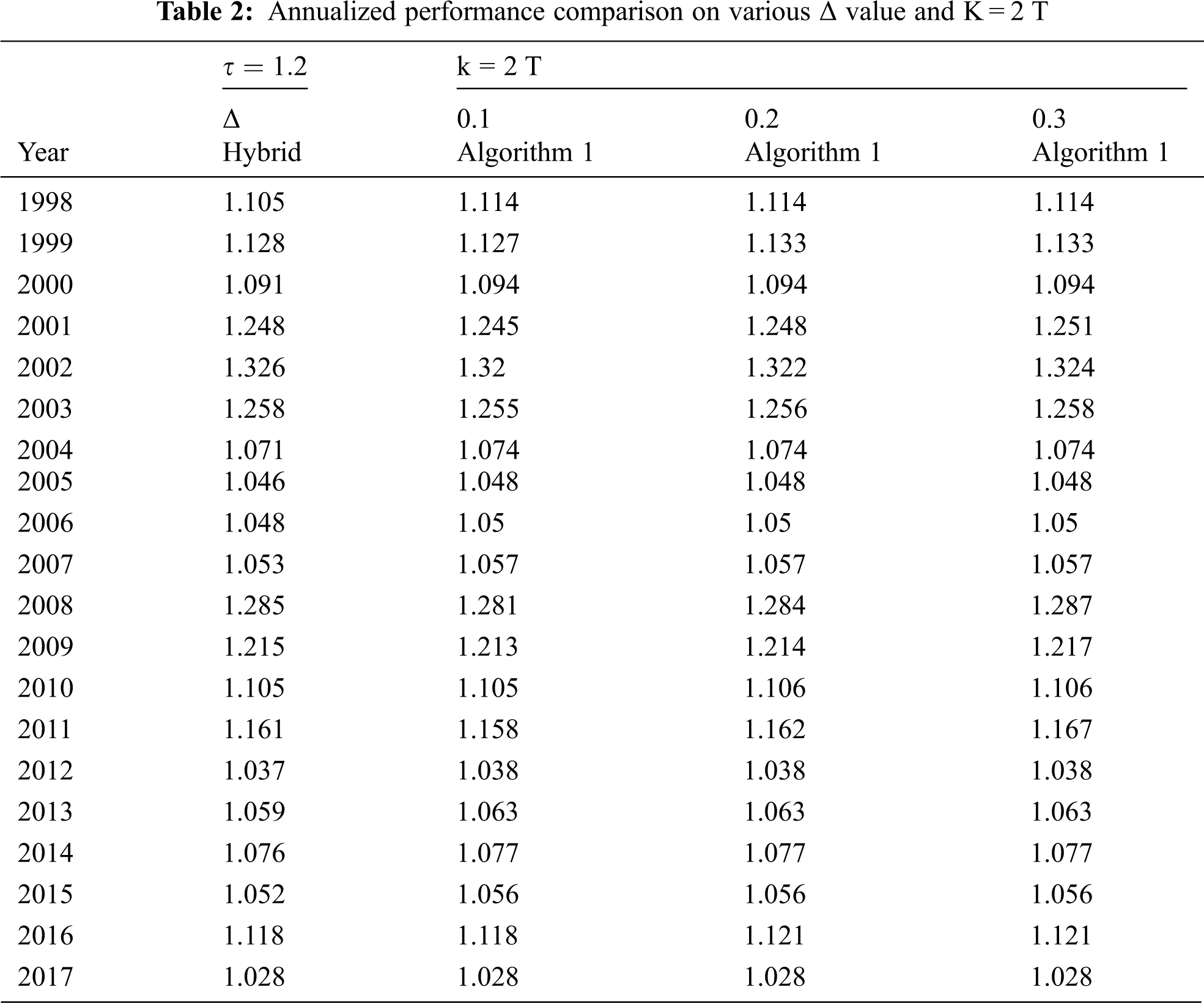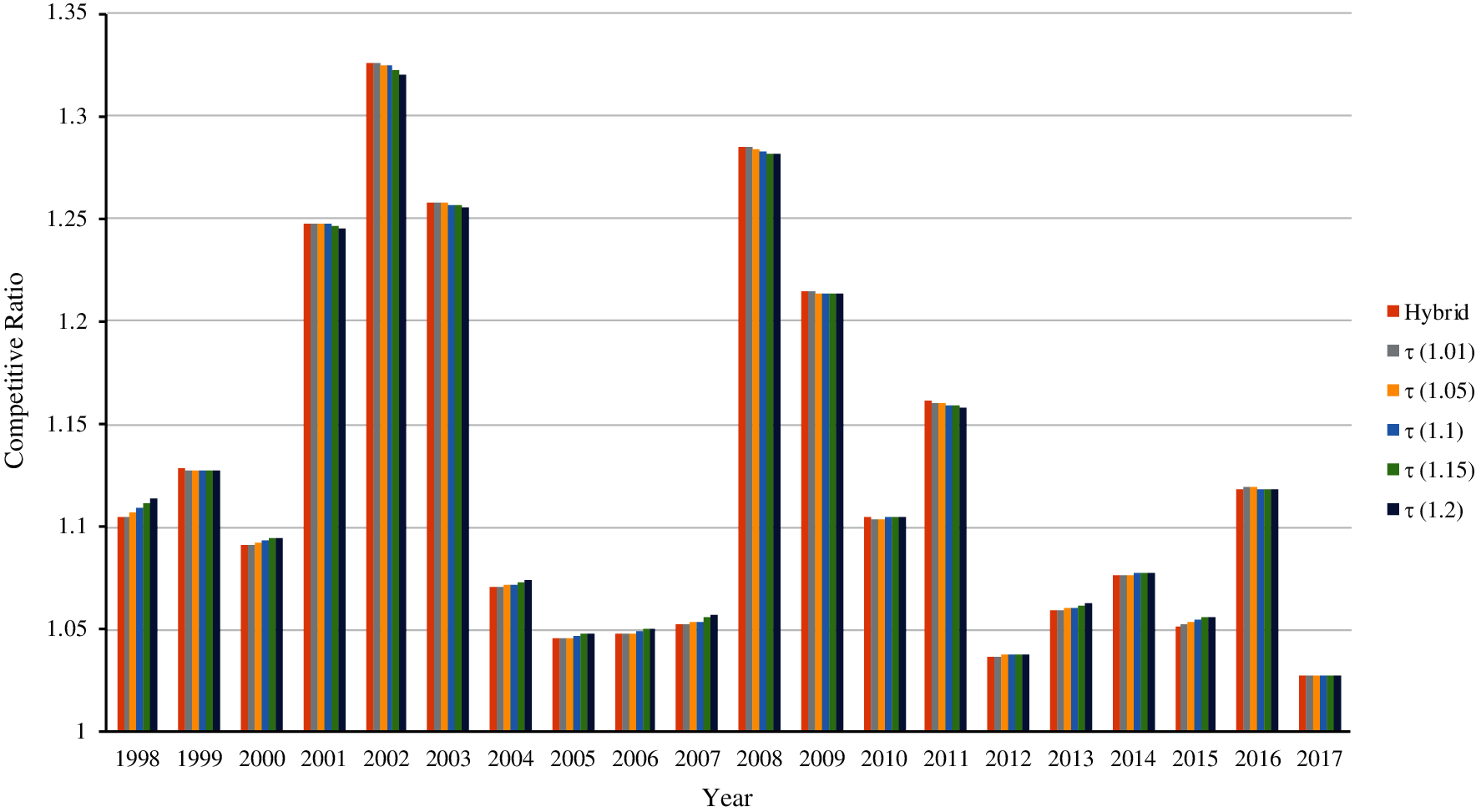[BACK]
 Intelligent Automation & Soft ComputingDOI:10.32604/iasc.2022.020715Article

Competitive Risk Aware Algorithm for k-min Search Problem

1Department of Computer Science and Information Technology, University of Engineering and Technology, Peshawar, Pakistan
2University of Jeddah, College of Computing and Information Technology at Khulais, Department of Information Technology, Jeddah, Saudi Arabia
3University of Jeddah, College of Computer Science and Engineering, Department of Software Engineering, Jeddah, Saudi Arabia
*Corresponding Author: Iftikhar Ahmad. Email: ia@uetpeshawar.edu.pk
Received: 03 June 2021; Accepted: 04 July 2021

Abstract: In a classical k-min search problem, an online player wants to buy k units of an asset with the objective of minimizing the total buying cost. The problem setting allows the online player to view only a single price quotation at each time step. A price quotation is the price of one unit of an asset. After receiving the price quotation, the online player has to decide on the number of units to buy. The objective of the online player is to buy the required k units in a fixed length investment horizon. Online algorithms are proposed in the literature for k-min search problem; however, these algorithms are risk averse in nature. We propose a risk aware k-min search algorithm which allows the online player to manage her risk level. The proposed algorithm is evaluated against the benchmark algorithm based on a real-world scenario using DAX30 data set. The proposed algorithm achieved up to 36.67% better results than the corresponding benchmark algorithm.

Keywords: Time series search; risk reward framework; online algorithms

1  Introduction

Time series search has received considerable attention from researchers in the recent past . Time series search involves searching for a minimum (or maximum) value in a time series where prices are revealed in an online manner. By online, we mean that at each time point, only one value is revealed and the player (user) has to decide immediately if the presented value will be the minimum (or maximum) in the whole time series, without the knowledge of future prices [3,5]. Likewise, the player cannot go in the past and accept a price that is already rejected by her. As the player has no knowledge of the future prices and cannot go back in the past to accept a rejected price, achieving an optimum (minimum) value is not possible. Therefore, the objective of the player is to achieve a value which is as close to the minimum value as possible [5,6]. Secretary search is one of the popular applications of time series search.

Time series search is categorized into min search and max search problems. In min search, the online player is searching for a minimum value, whereas in max search the online player is searching for a maximum price . In a general min search problem, the online player has to accept one price. If no such price is accepted in the first T - 1 days, the last offered price, offered at day T must be accepted . The general min search problem is akin to buying a single unit of an asset with the objective to minimize the buying cost. k-min search is an extension of general min search problem [6,7]. In the k-min search problem, the online player has to buy k units of an asset in a fixed length investment horizon. The objective is to minimize the buying cost of the required k units .

Rest of the paper is organized as follows. In Section 2, we present the preliminaries required to understand the work as well as the formal problem setting. Our proposed algorithm is presented in Section 3, along with derivation of the required formulae. Section 4 presents experimental settings based on various scenarios as well as the discussion on results. Section 5 concludes the work and present directions for future research.

2  Preliminaries and Problem Setting

In this section, we present the basic definitions forming the basis of this work, followed by the formal problem statement.

2.1 Preliminaries

Online Problem: In an online problem, the input is revealed to the algorithm in a serial fashion one at a time. Formally, let σ represent the input sequence such that σ =  σ(1), σ(2), …, σ(T) is the complete input sequence. In an online problem, at time point t  ∈ [1, T], an input σ(t) is revealed. The job of the online algorithm is to process the input σ(t) before σ(t^) is revealed. Note that t^>t .

Offline Problem: In an offline problem, the complete input sequence σ is revealed at the time point t = 1. The offline algorithm takes the decision based on all available information.

Generally, online algorithms are more challenging to design than offline algorithms. Online algorithms are evaluated using competitive analysis approach.

Competitive Analysis: Competitive analysis measure the performance of an online algorithm against an optimum offline algorithm. Let ON be an online algorithm for a cost minimization problem and OPT be an optimum offline algorithm for the same problem. Note that OPT represents the best possible solution for the problem. Let ON(σ) be the performance of online algorithm ON an input instance σ, where σ  ∈  Ψ. Note that Ψ represents the set of all possible input instances. The performance of optimum offline algorithm OPT on input instance σ  ∈  Ψ is represented by OPT(σ). Online algorithm ON is called c-competitive if for each input instance σ  ∈  Ψ ;

ON(σ)c.OPT(σ) (1)

2.2 Problem Setting

k-min search is an extension of general min search problem. In a k-min search problem, the objective of the player is to buy k units of an asset with minimum possible price. At each time point t  ∈ [1, T] the online player is offered a price qt  ∈ [m, M]. Note that m and M represents the minimum and maximum bounds of offered prices, i.e., all the price offers must be in this range. This information is necessary as otherwise it will not be possible to design an online algorithm with bounded competitive ratio . The online nature of the problem means that the player has access to the current time qt and does not know about future prices. After observing a price quotation qt, the online player has to decide on the number of units to buy without knowledge of the future prices. The game ends when the player buys the required number of k units or the last day is reached where the online player has to buy any remaining units at the last offered price qT.

3  Proposed Algorithm

We present our proposed algorithm as shown in Algorithm 1.According to the algorithm, the scheme is divided into two regimes; one when the forecast is true and second when it is false. When it is true the objective is to buy more than one unit. In fact, τ * j units are bought where τ is the tolerance level. When the forecast is false algorithms will buy ⌊j/τ⌋ units which are as fewer units as tolerance level allows.

Recall that the problem scope permits the online player to purchase multiple units of an asset. The determination of how many numbers of units to purchase is based on the extended price and tolerance level (τ). When the forecast is true, follow the Steps 6–8 and when it is false, follow Steps 10–12.

3.1 Computing qi and c

We consider a two-player game between an online player who wants to buy k units of an asset and a malicious adversary who wants to maximize the loss (competitive ratio) of the online player. We show that the malicious adversary has the ability impose a competitive ratio of at least c irrespective of the actions taken by the online player.

The online player has two options at each offered price. Either she refuses and look for a lower (better) price, or she accepts qt and purchase some units. Remember the aim of the online player is to ensure the competitive ratio not worse than c. While the opponent objective is to enforce the competitive ratio not less than c. The opponent (adversary) provides a price q1 , whenever the online player refuses this price, the opponent increases the price instantly to M and it remains there for the rest of the period. The online player purchases all the k units at M, resulting in commutative cost kM. Optimum offline algorithm purchases all the k units at q1 and thus accruing a cost of kq1 . The competitive ratio attained is c1 = kM/kq1 . If the online player accepts the price q1  and purchases one unit, the adversary decreases the price to q2 such that q2 < q1 (see Eq. (2)). Now once again, at q2 the online candidate has two options and on the basis of the selection made by her, the malicious adversary reacts and provides the next price. Whenever the candidate neglects q2 by declaring not to purchase any units, the opponent reacts by increasing the price to M. She obtains a cost of [q2+ (k1)M]  by purchasing one unit at q2 and the rest of (k  1) units at the maximum price M, while OPT purchases the required k units at q2 . OPT obtains a total cost of kq2 , and a competitive ratio of c2=q2+(k1)M/kq2 is attained by the online player. Whenever she accepts q2 and purchases one unit, the opponent decreases the price to q3 , and so on.

Similarly, if on a specific day the opponent offers the price qj and the candidate refuses to purchase any units. The opponent fixes the rest of the prices to M and remain there for the remaining investment period, whose consequence is a competitive ratio of cj=[q1+q2++(k(j1))M]/kqj .

If the online player continues accepting all reservation prices, the adversary provides a price in a succession q1,q2,.,qk,m . The online candidate purchases k units at a total cost of q1+q2+.+qk , while optimum offline algorithm OPT purchases all k units at m, ensuring a competitive ratio of ck+1=[q1+q2+.+qk]/km . The demonstrated scenario is summarized as, the reservation prices qj remain fixed for the opponent (for j  =  1 to k) such that the several competitive ratios cj (for j  =  1to k  +  1) are all equal. This common value is described by c. This signifies that regardless of the series of mechanism employed by the online player, she cannot attain a better competitive ratio than c. This outcomes in the (k  +  1) equations as shown in Eq. (2);

c=kMkq1c=q2+(k1)Mkq2c=q2+q3+(k2)Mkq3...c=q2+q3++qk+Mkqkc=q2+q3++qk+Mkm} (2)

Solving Eq. (2) for qi and c, we obtain;

qi=M[1(1(k1kc1))(1+(1kc1))i2] (3)

(1(k1)(kc1))(1+1(kc1))k(1mM)=0 (4)

4  Experimental Evaluation

We consider the hybrid algorithm proposed by Iqbal and Ahmad (2015) and our proposed risk aware algorithm (Algorithm 1). Each algorithm is executed on DAX30 yearly data. Competitive ratio for both algorithms is calculated on yearly data. It will be helpful to distinguish if a scheme is performing well on the basis of competitive ratio or vice versa.

4.1 Data Set

The experiments are performed on the real-world dataset of DAX30 from the year 1998 to 2017. DAX30 is the blue-chip stock market index consisting of 30 major German companies. We are considering daily closing prices.

4.2 Assumptions

We consider the following set of assumptions;

•   The prices are daily closing prices.

•   We assume that there is a forecast that in future the offered prices will reach a certain minimum level. It is not necessary that the forecast is always true, it can be false as well.

•   We assume a transaction fee of 2%.

•   We consider K  ={T/2,   T,   2T}, where K denotes the number of units to buy.

•   We assume tolerance level τ  ={1.01,   1.05,   1.10,   1.15,   1.20} , where tolerance level represents the risk level of the player. For example, if τ = 1.01 it means she will buy 1% more units than usual if the forecast is true.

•   We assume Δ = {0.10, 0.20, 0.30} where Δ determines the price from where the forecast will come true. It means that forecast will be true if the offered price reaches m(1 + Δ).

4.3 Settings

In order to perform a meaningful comparison between the state-of-the-art algorithm and our proposed algorithm, we consider the following scenarios covering various aspects of the problem settings.

Scenario 1: Tolerance τ = 1.2 and the number of units to buy k = T are fixed. Various values of Δ are considered, such that

Scenario 2: Tolerance τ = 1.2 and the number of units to buy are k = 2T are fixed. Various values of Δ are considered, such that Δ  =  {0.1, 0.2, 0.3}.

Scenario 3: Tolerance τ = 1.2 and Δ = 0.1 are fixed. The numbers of units k  =  {T/2, T and 2T} are considered.

Scenario 4: Number of units k = T and Δ = 0.1, the tolerance level τ  =  {1.01, 1.05, 1.10, 1.15, 1.2} is considered.

Scenario 5: Number of units k = 2T and Δ = 0.1, the tolerance level τ  =  {1.01, 1.05, 1.10, 1.15, 1.2} are considered.

4.4 Results and Discussions

The experiments are performed on the real-world dataset for various scenarios as discussed in Section 4.3. Hybrid and our proposed risk aware algorithm are executed on the dataset. The experiments performed using Scenario 1 produces the results as presented in Tab. 1. The first row of the table determines that the experiments are performed using tolerance level τ = 1.2 and the number of units to buy k = T.During experiments, tolerance (τ) and the number of units K are kept constant and the value of Δ = {0.10, 0.20, 0.30} varied. The results obtained shows that for some years’ hybrid gives better competitive ratio and at others the proposed risk aware scheme achieves superior performance.

The experiment performed using the Scenario 2 produces the results presented in Tab. 2. The first row of the table determines that the experiments are performed using tolerance level (τ) = 1.2 and number of units to buy k = 2T which means the player is buying two times more units than Scenario 1.Tolerance (τ) and the number of units k are kept constant and the Δ = {0.10, 0.20, 0.30} is modified. The results obtained shows that for some years’ hybrid gives better competitive ratio and at others the proposed risk aware scheme achieves superior performance.

The experiment performed using the Scenario 3 produces the results presented in Tab. 3. We kept τ and Δ constant and the number of units to buy K={T,T/2, 2T} varies.It is evident from the previous two scenarios that the results of proposed risk aware algorithm did not improve much. In Scenario 3 better results are achieved. In Scenario 1 the average performance percentage was 18.33% while in Scenario 2 the average performance percentage achieved was 23.33%, whereas this percentage is improved and raised to 36.67% in Scenario 3.

In Scenario 4 (see Fig. 1), we observed that the higher value of tolerance levels (τ) generates improved results. In year 2001 and 2002 risk-aware produced the least competitive ratio that is 1.245 and 1.322 respectively than hybrid. The results obtained show that for some years’ hybrid has given better competitive ratio and at others the proposed risk aware scheme achieves high performance.Figure 1: Performance comparison for K = T and Δ = 0.1 and varying values of τ

Scenario 5 relatively generates same results as we observed in Scenario 4. The only difference between the two scenarios are the number of units to buy such as k = 2T. The results (see Fig. 2) show that as we increase the tolerance level τ, risk aware generate improved results.Figure 2: Performance comparison for K = 2 T and Δ = 0.1 and varying values of τ

The outcomes of the experiments indicate that for several years’ hybrid provide better competitive ratio while for some the proposed risk aware algorithm attains high performance. Yet, the overall performance of the risk aware algorithm is inconsistent.

The risk aware algorithm shows good results when we have small value of Δ, higher value of τ and number of units k > T. The reason is that it is based on risk reward framework. The more the player takes risk the more she succeeds. It also happens that the more risk can lead to greater loss. For instance, It is observed from the results in year 2001, 2002 and 2003 that the competitive ratio is improved for risk aware algorithm over hybrid scheme and indeed reducing the total cost while in 2004 and 2007 the results show that the competitive ratio is at the higher side and risk aware lack in reducing the loss and indeed result in higher cost.

5  Conclusion

We presented an online k-min search scheme for the circumstance where an investor needs to purchase k units of an asset and furthermore suit the hazard and limiting the aggregate purchasing cost. The proposed scheme permits the online player (investor) to purchase in excess of one unit if the condition is good and less units if the condition is not reasonable. It accomplishes the better execution and preferable aggressive proportion over conventional approach.

The proposed scheme can be extended to other online algorithms for conversion problems with inter-related prices such as proposed by Schroeder et al. [14,15]. One of the limitations of the current work is the data snooping bias. It will be interesting to investigate the performance of the proposed algorithm against benchmark algorithm using bootstrap data. The bootstrap data can ensure that data snooping bias is addressed, and the results can then be generalized. An empirical study like the one conducted by Ahmad et al.  with risk aware algorithms can provide an interesting insight into crypto-currencies trading using algorithms. Likewise, a study investigating the effect of incomplete information on the performance of algorithms using the risk-reward framework can also be a research direction .

Funding Statement: The authors received no specific funding for this study.

Conflicts of Interest: The authors declare that they have no conflicts of interest to report regarding the present study.

References

1.  1.  T. Itoh and Y. Takei, “On the competitive analysis for the multi-objective time series search problem,” IEICE Transactions on Fundamentals of Electronics, Communications and Computer Sciences, vol. 102, no. 9, pp. 1150–1158, 2019.
2.  2.  S. P. Fung, “Optimal online two-way trading with bounded number of transactions,” Algorithmica, vol. 81, no. 11, pp. 4238–4257, 2019.
3.  3.  I. Ahmad, M. O. Ahmad, M. A. Alqarni, A. A. Almazroi and M. I. K. Khalil, “Using algorithmic trading to analyze short term profitability of bitcoin,” PeerJ Computer Science, vol. 7, pp. e337, 2021.
4.  4.  F. Li, “Two-way currency trading algorithms in the discrete setting,” in Proc. Int. Conf. on Algorithmic Applications in Management, pp. 169–178, Beijing, China Springer, 2019.
5.  5.  R. El-Yaniv, A. Fiat, R. M. Karp and G. Turpin, “Optimal search and one-way trading online algorithms,” Algorithmica, vol. 30, no. 1, pp. 101–139, 2001.
6.  6.  J. Iqbal and I. Ahmad, “Optimal online k-min search,” EURO Journal on Computational Optimization, vol. 3, no. 2, pp. 147–160, 2015.
7.  7.  J. Lorenz, K. Panagiotou and A. Steger, “Optimal algorithms for k-search with application in option pricing,” Algorithmica, vol. 55, no. 2, pp. 311–328, 2009.
8.  8.  W. Zhang, Y. Xu, F. Zheng and M. Liu, “Online algorithms for the general k-search problem,” Information Processing Letters, vol. 111, no. 14, pp. 678–682, 2011.
9.  9.  J. Iqbal, I. Ahmad, A. Shah and A. B. M. Asadullah, “Risk aware k-min search algorithm,” in Proc. 2019 IEEE 6th Int. Conf. on Engineering Technologies and Applied Sciences (ICETASKuala Lumpur, Malaysia, 2019.
10. 10. S. al-Binali, “A risk-reward framework for the competitive analysis of financial games,” Algorithmica, vol. 25, no. 1, pp. 99–115, 1999.
11. 11. W. Zhang, E. Zhang and F. Zheng, “Online two stage k-search problem and its competitive analysis,” International Journal of Foundations of Computer Science, vol. 27, no. 6, pp. 653–663, 2016.
12. 12. G. Schmidt, “Competitive analysis of bi-directional non-preemptive conversion,” Journal of Combinatorial Optimization, vol. 34, no. 4, pp. 1096–1113, 2017.
13. 13. M. Schwarz, “Lower and upper bounds for the discrete bi-directional preemptive conversion problem with a constant price interval,” Algorithms, vol. 13, no. 2, pp. 1–12, 2020.
14. 14. P. Schroeder, R. Dochow and G. Schmidt, “Optimal solutions for the online time series search and one-way trading problem with interrelated prices and a profit function,” Computers & Industrial Engineering, vol. 119, pp. 465–471, 2018.
15. 15. P. Schroeder and I. Kacem, “Optimal cash management with uncertain, interrelated and bounded demands,” Computers & Industrial Engineering, vol. 133, pp. 195–206, 2019.
16. 16. W. Wang, L. Wang, Y. Lan and J. X. Zhang, “Competitive difference analysis of the one-way trading problem with limited information,” European Journal of Operational Research, vol. 252, no. 3, pp. 879–887, 2016.This work is licensed under a Creative Commons Attribution 4.0 International License, which permits unrestricted use, distribution, and reproduction in any medium, provided the original work is properly cited.